Next: Visualization results part two Up: Interactivity Previous: CGI scripts

# Do it yourself null geodesic

By using the interactive form below, you can create a 3D scene with your own null geodesics. This is a very good way of getting a feeling of how light rays move around a rotating black hole.

#### Black hole properties

M   =     (mass)
a/M   =     (measure of rotation,)

#### Initial position of photon

r   =     (you must have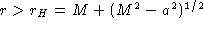)=     [radians]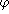=     [radians] (unimportant, since there is symmetry around the z-axis)
t   =     (unimportant, since the metric is time independent)

Note: You can specify(pi) as   !pi   in the normal IDL fasion.

#### Initial direction of photon

dr   =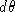=     [radians]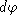=     [radians]
Note: Here, a null geodesic is obtained by solving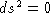for dt. Therefore, the user cannot specify dt.

#### Affine parameter control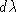=     (affine parameter step size)
# steps   =     (max 10,000)

#### 3D scene format

Inventor
VRML

Note: you have to manually switch on "Headlight" (in the "View" menu) in WebSpace to be able to see the 3D widgets below. In ivview there seems to be light everywhere.

#### Drawing style of geodesic

Line
Points

Note: I think that Line looks the best, but Points allows you too see (more clearly) the spacing between the points. Try sending the photon directly towards a nonrotating black hole, e.g. a/M=0, dr=-0.5,=0, and=0.

#### Color of geodesic

R   =     G   =     B   =

#### Coordinate system widget

• Enabled   or   Disabled
• Axis length   =
• Label x axis with 3D letter "x": yes   or   no

Note: the x, y, and z axis are red, green, and blue, respectively, and have a transparency of 0.25. If you cannot view color, you should enable the x axis labeling, and then find the y and z axis using the right hand rule.

#### Horizon widget

• Enabled   or   Disabled
• Radius: r   =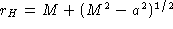or   r   =
• Color:   R   =     G   =     B   =
• Transparency   =
Note: you can of course get a black horizon instead of a yellow one by setting R=G=B=0 !

#### Static Limit / Ergosphere widget

• Enabled   or   Disabled
• Color:   R   =     G   =     B   =
Note: the static limit is given by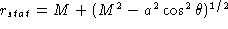.
It is shown as a wire mesh.

#### Submit!(be patient!) or
Note: when your browser says "Connect: Host www.astro.ku.dk contacted. Waiting for reply...", my machine is actually calculating the null geodesic you specified.
Typical calculation time: 10 seconds.

Web Exhibition: Null Geodesics Around a Kerr Black HoleBo Milvang-Jensen (milvang@astro.ku.dk)
Mon Jun 17 11:54:08 MDT 1996